# 5.9.4 Electrolysis (Structured Questions)

Question 1:
Diagram 1 shows the setting up of apparatus in an experiment.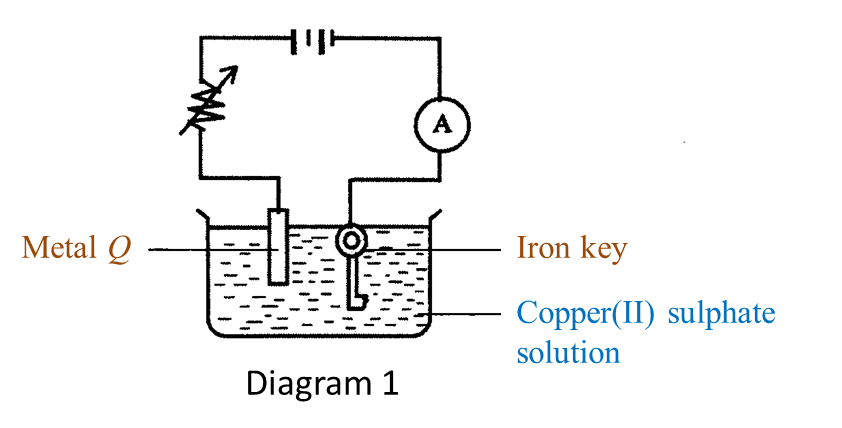(a) Name the process in Diagram 1. [1 mark]

(b)(i) Name metal Q. [1 mark]

(ii) What happens to metal Q during the process in Diagram 1? [1 mark]

(c) Which metal functions as the cathode? [1 mark]

(d)(i) What will happen to the iron key at the end of the experiment? [1 mark]

(ii) State one method to get a good result in (d)(i) [1 mark]

Answer:
(a) Electroplating

(b)(i) Copper
(b)(ii) Metal Q dissolves in the copper(II) sulphate solution to form copper ions and becomes thinner.

(c) Iron key.

(d)(i) The surface of the iron key will be coated with a brown layer of copper.
(d)(ii) The surface of the metal to be plated must be cleaned with sandpaper before electrolysis begins.

# 5.9.3 Application of Reactivity Series of Metals (Structured Questions)

Question 1:
Diagram below shows the extraction of tin ore at high temperature in a blast furnace.(a) Name two elements in R. [2 marks]

(b) State one reason why carbon is a suitable element to use in the extraction of tin ore from R? [1 mark]

(c) Name gas S. [1 mark]

(d) What is the function of limestone in this process? [1 mark]

(e) Name product T. [1 mark]

Answer:
(a) Tin, oxygen

(b)
As tin is less reactive than carbon, carbon can be used to reduce the tin ore from R.

(c)
Carbon dioxide.

(d)
The limestone is decomposed to produce quicklime (calcium oxide) which combines with impurities such as silica to form slag.

(e)
Slag

# 5.9.2 The Reactivity Series of Metals (Structured Questions)

Question 1:
Diagram 1.1 and Diagram 1.2 show an experiment to study the reactivity of metals with water.(a) Observe Diagram 1.1. State the volume of gas collected.
Write down your answer in Table below. [1 mark]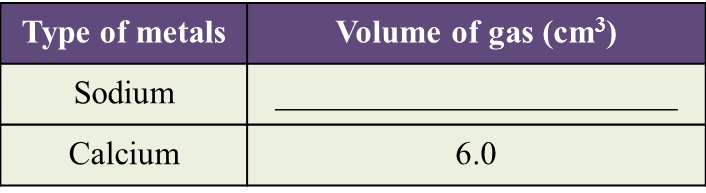(b) Based on the result in Table above, state one inference. [1 mark]

(c) State the variables in this experiment.
(i) Constant variable [1 mark]
(ii) Responding variable [1 mark]

(d) Based on this experiment, mark (\/) the metal which is more reactive. [1 mark]

Answer:
(a)(b)
A reactive metal can displace more gas from water.

(c)(i)
The mass of the metal / The time taken

(c)(ii)
The volume of gas collected

(d)

# 7.7.2 Respiratory Structure and Breathing Mechanisms in Humans and Animals (Structured Question 1 & 2)

[adinserter block="3"]
Question 1:
Figure I represents a model of the human lungs in the respiratory mechanism.(a)
Based on the model of the lungs in Figure I, what are the equivalent structures to the glass tube and the bell jar in the human respiratory system?
Glass tube: __________
Bell jar: ____________
(2 marks)

(b)(i)
The thin rubber sheet represents the diaphragm in the human respiratory system.
What is the function of the thin rubber sheet in the model of the lungs? (1 mark)

(ii)
The balloons represent the human lungs.
Explain one characteristic of the balloons which is similar to the human lungs. (2 marks)
[adinserter block="3"]

(c)(i)
The string in the model of the lungs is pulled down.
Draw the changes to the thin rubber sheet and the balloons in Figure II below. (1 mark)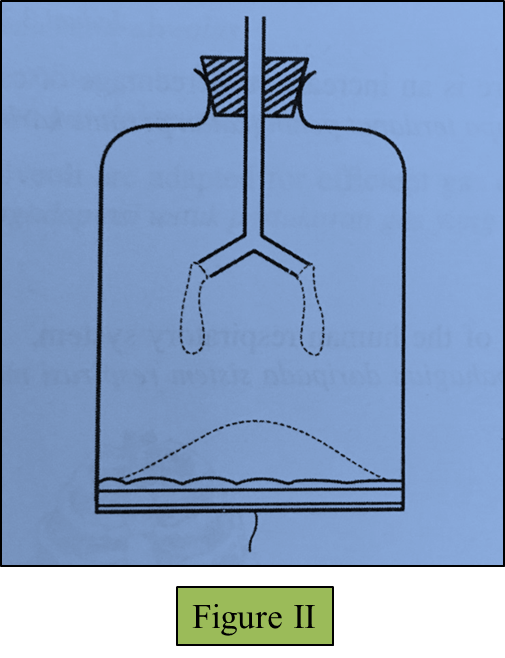(ii)
Observe your drawing in (c)(i).
Explain the relationship between the changes in the model of the lungs you have drawn and the real human respiratory system. (3 marks)
[adinserter block="3"]

(d)(i)
The percentage of oxygen and carbon dioxide gases in inspired and expired air is determined by using the J-tube.
Why is the end of the J-tube dipped in potassium hydroxide solution and then followed by potassium pyrogallol solution? (1 mark)

(ii)
Table below shows the result of a study on the content of inspired and expired air.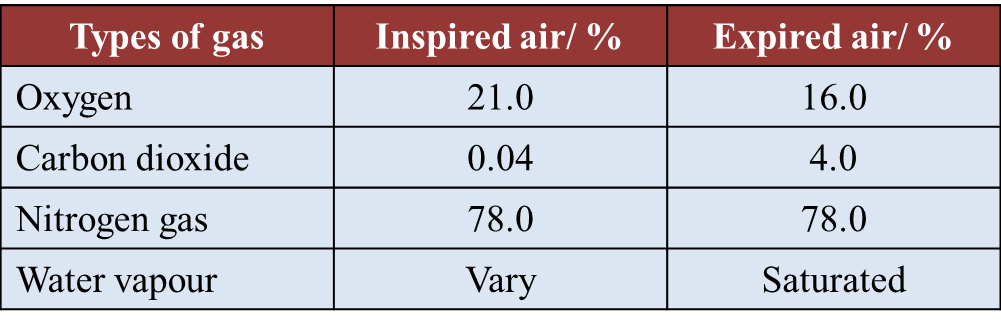Explain why there is an increase in percentage of carbon dioxide in the expired air. (2 marks)

[adinserter block="3"]
Answer:
(a)
Glass tube: Bronchi/ trachea
Bell jar: Rib cage/ ribs

(b)(i)
To control the volume/ pressure of the air in the bell jar

(b)(ii)
Balloons are elastic and can expand and contract

(c)(i)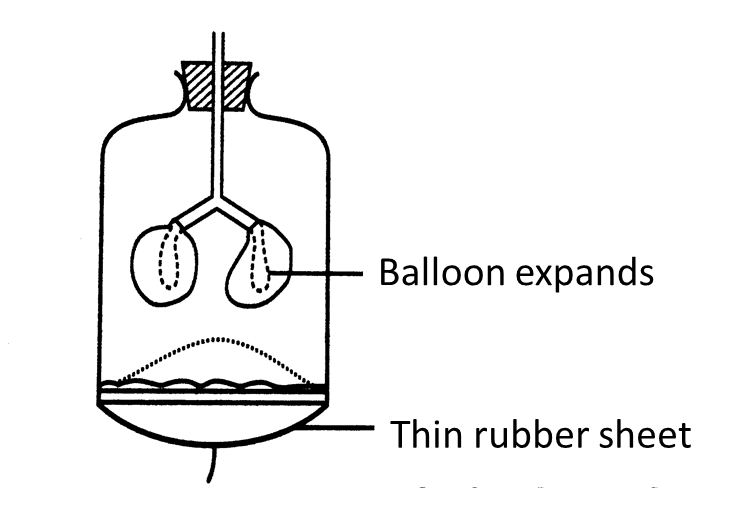[adinserter block="3"]
(c)(ii)
The diaphragm muscles contract causing the volume of the thoracic cavity to increase. This causes the pressure in the lungs to decrease. Air from the outside is forced in.

(d)(i)
Potassium hydroxide solution absorbs carbon dioxide only whereas potassium pyrogallol solution absorbs both oxygen and carbon dioxide.

(d)(ii)
Carbon dioxide is the product of cellular respiration in the body. Carbon dioxide diffuses out of the cells to be transported to the lungs.

[adinserter block="3"]

Question 2:
(a) Sugar is oxidized during respiration.
Explain the process. (4 marks)

(b)
After an athlete finished running a race, his breathing is still fast and deep for several minutes. (6 marks)
Explain why.

(c)
State the similarities and differences between the human and the grasshopper’s respiratory system based on the structural adaptation to maximize the rate of gaseous exchange. (10 marks)

[adinserter block="3"]
Answer:
(a)
- During respiration, oxygen taken in is transported by the blood circulatory system to the body cells.

- In the cells, sugar (glucose molecules) are oxidized by the oxygen to release energy in the form of ATP.

- Other products of this oxidation process are carbon dioxide and water.
[adinserter block="3"]

(b)

- He needs to breathe in fast and deep in order to inhale more oxygen.

- This is because during running, the rate of oxygen being used by the muscles exceeds the amount of oxygen supplied by the blood.

- The muscles are in a state of oxygen deficiency.

- An oxygen debt is incurred.

- The muscles obtain the extra energy (ATP) from anaerobic respiration, because oxygen is not available.

- The excess oxygen inhaled is used by the body to oxidise the accumulated lactic acid to carbon dioxide and water.

- When all the lactic acid is removed, the oxygen debt is paid off.
[adinserter block="3"]

(c)

Similarities:
- Both respiratory systems have structures which have a large surface area to volume ratio for efficient gaseous exchange.

- The cells that lining the respiratory structures are thin. This allows gas diffusion to take place efficiently.

- The surfaces for gaseous exchange are constantly moist because they are covered by a film of water which allows the respiratory gases to dissolve in it.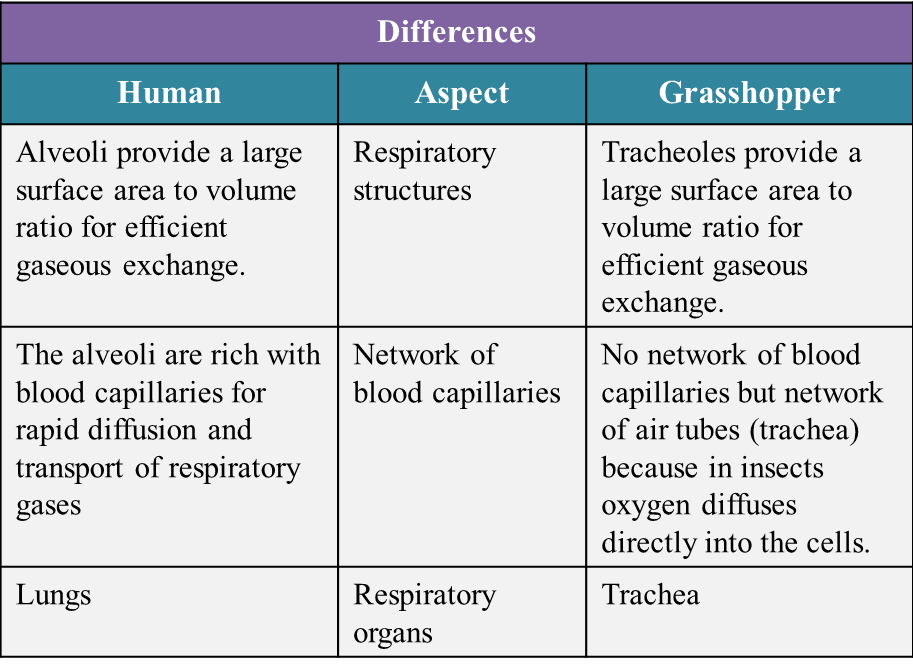# 5.9.1 Heat Change in Chemical Reactions (Structured Questions)

Question 1:
Diagram 1.1 and Diagram 1.2 show an experiment to study the heat change in chemical reactions at room temperature.(a) Based on Diagram 1.1, what is your observation about the change in temperature?
Mark (\/) for your answer in Table below. [1 mark]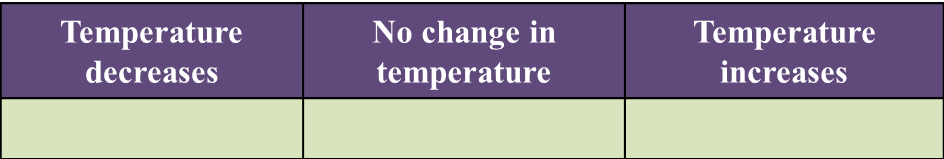(b) What is the final reading for the thermometer in Diagram 1.2? [1 mark]

(c) State the variables in this experiment.
(i) Constant variable [1 mark]
(ii) Responding variable [1 mark]

(d) State one hypothesis for this experiment. [1 mark]

Answer:
(a)(b)
35 oC

(c)(i)
The amount of water or initial temperature

(c)(ii)
The final temperature

(d)
In a chemical reaction, heat is given out causing a rise in temperature or is absorbed causing a drop in temperature.

# 7.7.1 The Respiratory Process in Energy Production (Structured Question 1 & 2)

[adinserter block="3"]
Question 1:
Diagram below shows a type of organelle found in muscle cells.
A biochemical reaction occurs in the organelle.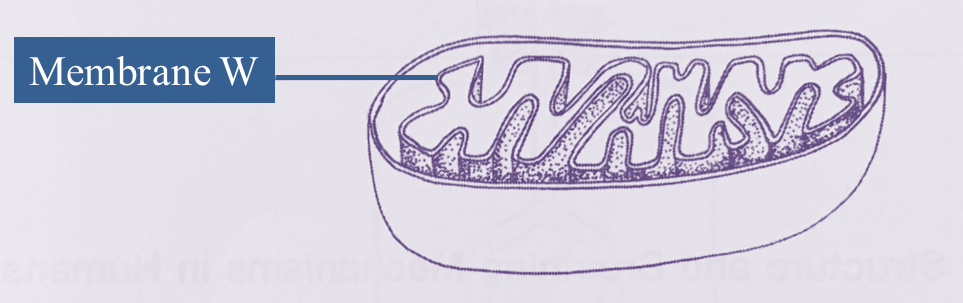(a)(i)
Name this organelle. [1 mark]

(ii)
Explain why membrane W is in the form of numerous folded layers. [2 marks]
[adinserter block="3"]

(b)
The biochemical reaction that occurs in this organelle is summarized as follows:(i) Name gas R. [1 mark]

(ii)
Name product S. [1 mark]

(iii)
Explain why the muscle cell has a large number of these organelles. [2 marks]

(iv)
If the blood sugar level is lower than the normal range, the biochemical reaction in this organelle can still occur.
Explain how. [3 marks]
[adinserter block="3"]

(c)
State two differences on the biochemical reaction occurring between muscle cell and yeast cell in the absence of gas R. [2 marks]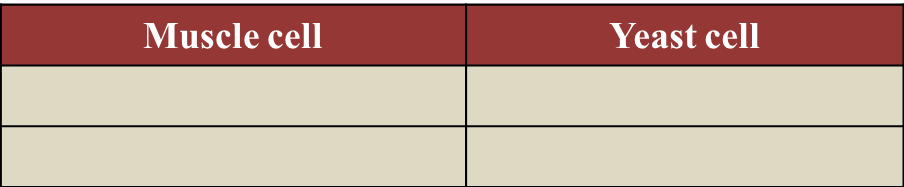[adinserter block="3"]
Answer:
(a)(i)
Mitochondrion

(a)(ii)
Membrane W is in the form of numerous folded layers to increase total surface area, for the efficiency of cellular respiration.

(b)(i)
Gas R is oxygen

(b)(ii)
Product S is water

(b)(iii)
Muscle cells need a large number of mitochondrion as an energy ATP supply in order to oxidise glucose in aerobic respiration.
[adinserter block="3"]

(b)(iv)
- If the blood sugar is lower than the normal range, less energy is produced.
- Pancreas secretes glucagon to convert glycogen to glucose.
- Cellular respiration occurs.

(c)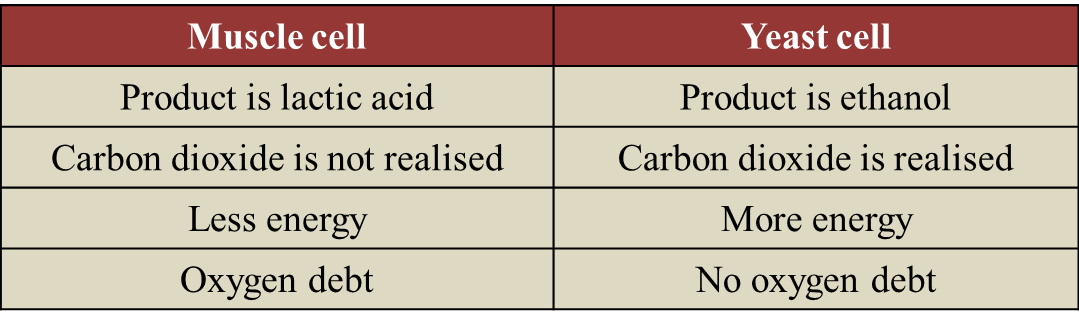[adinserter block="3"]